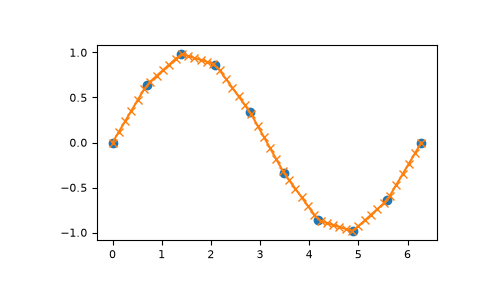# numpy.interp¶

`numpy.``interp`(x, xp, fp, left=None, right=None, period=None)[source]

One-dimensional linear interpolation for monotonically increasing sample points.

Returns the one-dimensional piecewise linear interpolant to a function with given discrete data points (xp, fp), evaluated at x.

Parameters
xarray_like

The x-coordinates at which to evaluate the interpolated values.

xp1-D sequence of floats

The x-coordinates of the data points, must be increasing if argument period is not specified. Otherwise, xp is internally sorted after normalizing the periodic boundaries with `xp = xp % period`.

fp1-D sequence of float or complex

The y-coordinates of the data points, same length as xp.

leftoptional float or complex corresponding to fp

Value to return for x < xp, default is fp.

rightoptional float or complex corresponding to fp

Value to return for x > xp[-1], default is fp[-1].

periodNone or float, optional

A period for the x-coordinates. This parameter allows the proper interpolation of angular x-coordinates. Parameters left and right are ignored if period is specified.

New in version 1.10.0.

Returns
yfloat or complex (corresponding to fp) or ndarray

The interpolated values, same shape as x.

Raises
ValueError

If xp and fp have different length If xp or fp are not 1-D sequences If period == 0

Warning

The x-coordinate sequence is expected to be increasing, but this is not explicitly enforced. However, if the sequence xp is non-increasing, interpolation results are meaningless.

Note that, since NaN is unsortable, xp also cannot contain NaNs.

A simple check for xp being strictly increasing is:

```np.all(np.diff(xp) > 0)
```

Examples

```>>> xp = [1, 2, 3]
>>> fp = [3, 2, 0]
>>> np.interp(2.5, xp, fp)
1.0
>>> np.interp([0, 1, 1.5, 2.72, 3.14], xp, fp)
array([3.  , 3.  , 2.5 , 0.56, 0.  ])
>>> UNDEF = -99.0
>>> np.interp(3.14, xp, fp, right=UNDEF)
-99.0
```

Plot an interpolant to the sine function:

```>>> x = np.linspace(0, 2*np.pi, 10)
>>> y = np.sin(x)
>>> xvals = np.linspace(0, 2*np.pi, 50)
>>> yinterp = np.interp(xvals, x, y)
>>> import matplotlib.pyplot as plt
>>> plt.plot(x, y, 'o')
[<matplotlib.lines.Line2D object at 0x...>]
>>> plt.plot(xvals, yinterp, '-x')
[<matplotlib.lines.Line2D object at 0x...>]
>>> plt.show()
```Interpolation with periodic x-coordinates:

```>>> x = [-180, -170, -185, 185, -10, -5, 0, 365]
>>> xp = [190, -190, 350, -350]
>>> fp = [5, 10, 3, 4]
>>> np.interp(x, xp, fp, period=360)
array([7.5 , 5.  , 8.75, 6.25, 3.  , 3.25, 3.5 , 3.75])
```

Complex interpolation:

```>>> x = [1.5, 4.0]
>>> xp = [2,3,5]
>>> fp = [1.0j, 0, 2+3j]
>>> np.interp(x, xp, fp)
array([0.+1.j , 1.+1.5j])
```